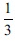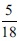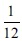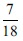# User ForumSubject :IMO    Class : Class 8

If a die is rolled two times, then find the probability of getting

 Column - I Column - II (i) Multiple of 2 on first die and multiple of 4 on second die. (A)(ii) Sum of number obtained on both the die as multiple of 3. (B)(iii) Number on the first die is divisible by number on second die. (C)(iv) Sum of the numbers on both die is divisible by the number on second die. (D)A(i) → (A); (ii) → (B); (iii) → (C); (iv) → (D)
B(i) → (C); (ii) → (A); (iii) → (D); (iv) → (B)
C(i) → (A); (ii) → (C); (iii) → (B); (iv) → (D)
D(i) → (C); (ii) → (A); (iii) → (D); (iv) → (D)